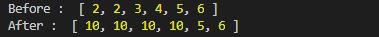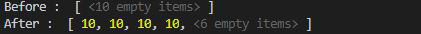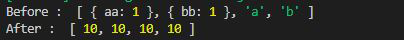Skip to content
Related Articles
Lodash _.fill() Method
• Last Updated : 29 Jul, 2020

Lodash is a library that works on the top of underscore.js. It proves to be very handy when it is used with objects, arrays, dictionaries and strings.
The lodash.fill() method is used to fill a set of values into the array in a given range.

Syntax:

`lodash.fill(array, value, startIndex, endIndex)`

Parameters:

• Array: It is the original array that is to be filled with certain values.
• Value: Value to be filled in the array.
• startIndex: It is the index from where value is to be filled.
• endIndex: It is the index to which values are to be filled in the array.

Note:

• All index less then endIndex are included except endIndex.
• Changes are done in the original array.

Return Value: It return the array.

Example 1:

## Javascript

 `// Requiring the lodash library``let lodash = require(``"lodash"``);`` ` `// Original array``let array = [2, 2, 3, 4, 5, 6]`` ` `// Printing original array ``console.log(``"Before : "``, array)`` ` `// Using fill() method to replace``// values in range (0, 4]``lodash.fill(array, 10, 0, 4)`` ` `// Printing original array again ``console.log(``"After : "``, array)`

Output:Example 2: When empty array is given.

## Javascript

 `// Requiring the lodash library``let lodash = require(``"lodash"``);`` ` `// Original array``let array = Array(10)`` ` `// Printing original array ``console.log(``"Before : "``, array)`` ` `// Using fill() method to add``// values in range (0, 4]``lodash.fill(array, 10, 0, 4)`` ` `// Printing original array again ``console.log(``"After : "``, array)`

Output:Example 3: when endIndex is greater then the size of array.

## Javascript

 `// Requiring the lodash library``let lodash = require(``"lodash"``);`` ` `// Original array``let array = [{ ``"aa"``: 1 }, ``        ``{ ``"bb"``: 1 }, ``"a"``, ``"b"``]`` ` `// Printing original array ``console.log(``"Before : "``, array)`` ` `// Using fill() method to replace``// values in range (0, 10] greater``// then size of array``lodash.fill(array, 10, 0, 10)`` ` `// Printing original array again ``console.log(``"After : "``, array)`

Output:My Personal Notes arrow_drop_up